+
Common Core: S.ID.6a S.ID.6c

# Finding the Least-squares Line

Author: Sophia Tutorial
##### Description:

Calculate the slope and y-intercept for a least-squares line.

(more)

Sophia’s self-paced online courses are a great way to save time and money as you earn credits eligible for transfer to many different colleges and universities.*

No credit card required

37 Sophia partners guarantee credit transfer.

299 Institutions have accepted or given pre-approval for credit transfer.

* The American Council on Education's College Credit Recommendation Service (ACE Credit®) has evaluated and recommended college credit for 32 of Sophia’s online courses. Many different colleges and universities consider ACE CREDIT recommendations in determining the applicability to their course and degree programs.

Tutorial
what's covered
This tutorial is going to teach you how to find the least-squares line of a data set. Our discussion breaks down as follows:

1. Discussing the Least-Squares Line
2. Calculating the Least-Squares Line

## 1. Discussing the Least-Squares Line

Recall that the least-squares line is a best-fit line that is found through a process of minimizing the sum of the squared residuals. The general form for a least-squares equation is: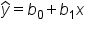In this equation, b0 is the y-intercept and b1 is the slope.

For a given data set, the least-squares line will always pass through the point (x̅, ȳ), where x-bar (x̅) is the mean of the explanatory data and y-bar (ȳ) is the mean of the response data.

The slope can be found using the following formula:

formula
Slope of Least Squares Line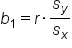The slope, b1, is found by multiplying the correlation coefficient by the ratio of the standard deviation of the y-data to the standard deviation of the x-data.

We can use these pieces of information to find the y-intercept and then create the least-square line equation.

terms to know

Least-Squares Line
A best-fit line that is found through a process of minimizing the sum of the squared residuals
X-bar (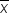)
The average x value for a sample
Y-bar (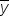)
The average y value for a sample

## 2. Calculating the Least-Squares Line

Look at airfare prices for certain destinations from the Minneapolis/St. Paul Airport. Boston is 1,266 miles from St. Paul, and it has an airfare of \$263, and so forth.

Destination Miles Airfare
Boston 1,266 263
Charleston 1,294 306
Chicago 407 128
Denver 834 212
Detroit 611 261

The scatter plot for this data looks like this: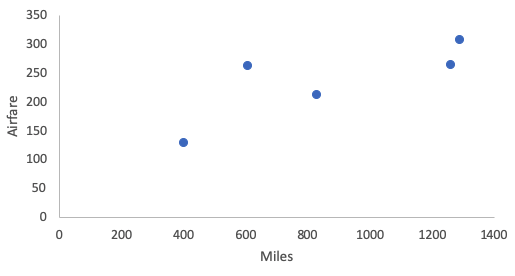We need to find a least-squares line that incorporates this data. The explanatory variable, x, will be miles and the predicted response variable, y-hat, will be airfare, so we can write the following equation to start: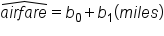So we need to find the slope and the y-intercept. To begin, we can use Excel to calculate the mean and standard deviation of both the x and y data. Type the data into an Excel spreadsheet and use the function "=AVERAGE" to calculate the mean and "=STDEV.S" to calculate the standard deviation.

 Miles Airfare 1,266 263 1,294 306 407 128 834 212 611 261 882.4 234 393 68 r = 0.794

In this scenario, miles is the explanatory x-variable, and airfare is the response y-variable. So the average miles, x̅, is 882.4 with a standard deviation, sx, of 393. The mean airfare, ȳ, is \$234 per ticket with a standard deviation, sy, of \$68.

We can also find the correlation easily with Excel. Use the function "=CORREL", highlight both the x- and y-data, and find the correlation coefficient of 0.794.

The slope of the line is equal to the correlation times the standard deviation of the response y-value, over the standard deviation of the explanatory x-value. Since you have these three values, all you have to do is plug them into the slope formula: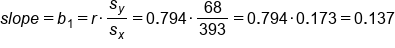The slope is going to be 0.794 times 68 over 393. The result of that is 0.137. So, what is that 0.137? That's the change in y, airfare in dollars, over a change in one of the miles. It's about 13.7 cents per mile.

Going back to the equation of the best-fit line, we still need to find the remaining information. We just found the slope, b1, is \$0.137 per mile. We still need to find the y-intercept, b0. We don't know this value, however, we do know a value for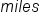and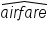. We know the average number of miles, x̅, and the average value of airfare, ȳ. Airfare is predicted to be \$234 when the miles is 882.4. Substitute this information into the equation and solve for the y-intercept, b0.You get 113.11 for b0 so put that all together with the slope to create a least-squares line: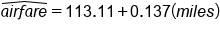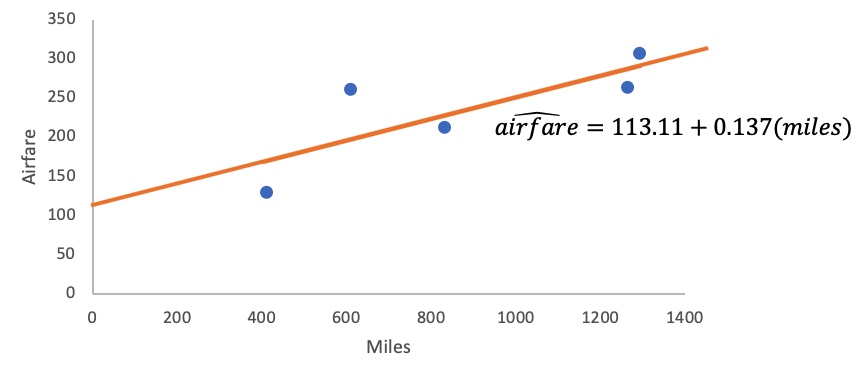Once it is graphed, it does appear to go right through the pack of points like it's supposed to.

try it
You can also use a spreadsheet. In Excel, the easiest way to do this is to highlight your data and create a chart that is a scatter plot. When you do this, you have to actually right-click or control-click onto the data points themselves so that they are highlighted. Click "Add Trendline." Under Options, click "Display Equation." Essentially it’s the same idea. Don't get too frustrated because technology can rescue you here. Especially for larger data sets, finding this by hand can be difficult.

summary
Calculation of the least-squares line involves two key facts: First, the point (x bar, y bar)--mean of explanatory variable, mean of response variable--is a point on the line; and second, that the slope is a calculable value from the correlation and the standard deviations that you have. You learned about the least-squares line and calculating the least-squares line, and you used all of these values plus correlation in order to find it.

Good luck!

Source: Adapted from Sophia tutorial by Jonathan Osters.

Terms to Know
Least-Squares Regression Line

The line of best fit, according to the method of Least-Squares.

x-bar

The mean of the explanatory variable.

y-bar

The mean of the response variable.

Formulas to Know
Slope of Least Squares LineRating